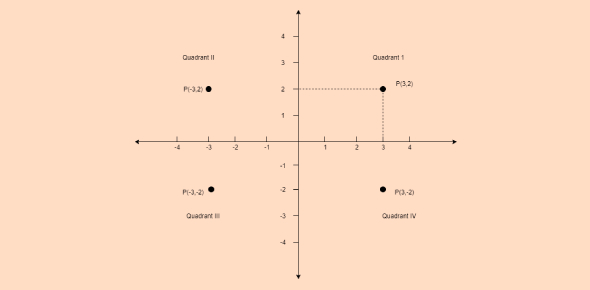# JEE Main Mathematics: Co-ordinate Geometry Quiz!

10 Questions | Attempts: 110
ShareSettings.

• 1.
If a, b, c are related by 9a2 + 16b2 – 16c2 + 24ab = 0then the greatest distance between any two lines of the family of lines ax + by + c = 0 is:
• A.

4/3

• B.
• C.
• D.
• 2.
If a1, a2, a3 as well as b1, b2, b3 are in G.P. with the same common ratio, then the points (a1, b1), (a2, b2) and (a3, b3):
• A.

Are vertices of a triangle

• B.

Lie on parabola

• C.

Lie on a circle

• D.

Lie on a straight line

• 3.
What will be the equation of the straight line passing from the point (–3, 5) and making intercept of length between the lines x + 3y +1 = 0 and x + 3y – 1 = 0?
• A.

X + 2 = 0

• B.

3x – y + 13 = 0

• C.

3x + 4y =18

• D.

4x + 3y = 12

• 4.
The line y = x meets y = k at: (Given k ≤ 0)
• A.

One point

• B.

Two points

• C.

Three points

• D.

Zero points

• 5.
The angle between the pair of straight lines y2sin2θ – xysin2θ + x2 (cos2θ – 1) = 0 is:
• A.
• B.
• C.
• D.
• 6.
The equation: x2y2 – 2xy2 – 3y2 – 4x2y + 8xy + 12y = 0; represents:
• A.

A pair of lines

• B.

A circle and pair of lines

• C.

Four lines

• D.

None of these

• 7.
Circles x2 + y2 + 2ax + c = 0 and circle x2 +y2 +2by + c = 0, touches each other then which of the following is correct?
• A.

1/a + 1/b = 1/c

• B.
• C.
• D.
• 8.
The number of common tangents to the circle x2 + y2 – 4 = 0 and x2 + y2 – 6x – 8y – 24 = 0 is:
• A.

0

• B.

1

• C.

3

• D.

• 9.
If the two circles x2 + y2 = r2 and x2 + y2 – 10x + 16 = 0 intersect each other at some real and distinct points then which of the following is true?
• A.

2 < r < 8

• B.

R = 2

• C.

R < 3

• D.

R > 5

• 10.
Lines x2 – 8x + 12 = 0 and y2 – 14y + 45 = 0 form a square. What will be the center of the circle inscribed in the square?
• A.

(4, 3)

• B.

(3, 4)

• C.

(4, 7)

• D.

(7, 4)

## Related TopicsBack to top
×

Wait!
Here's an interesting quiz for you.